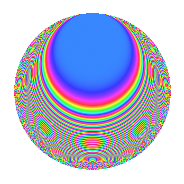# Properties

 Label 96.2.nLevel 96 Weight 2 Character orbit n Rep. character $$\chi_{96}(13,\cdot)$$ Character field $$\Q(\zeta_{8})$$ Dimension 32 Newform subspaces 1 Sturm bound 32 Trace bound 0

# Related objects

## Defining parameters

 Level: $$N$$ = $$96 = 2^{5} \cdot 3$$ Weight: $$k$$ = $$2$$ Character orbit: $$[\chi]$$ = 96.n (of order $$8$$ and degree $$4$$) Character conductor: $$\operatorname{cond}(\chi)$$ = $$32$$ Character field: $$\Q(\zeta_{8})$$ Newform subspaces: $$1$$ Sturm bound: $$32$$ Trace bound: $$0$$

## Dimensions

The following table gives the dimensions of various subspaces of $$M_{2}(96, [\chi])$$.

Total New Old
Modular forms 72 32 40
Cusp forms 56 32 24
Eisenstein series 16 0 16

## Trace form

 $$32q + O(q^{10})$$ $$32q - 8q^{10} - 16q^{12} - 32q^{14} - 8q^{16} - 8q^{18} - 32q^{20} - 8q^{22} - 16q^{23} - 8q^{24} + 40q^{26} + 40q^{28} - 48q^{31} + 40q^{32} + 40q^{34} - 48q^{35} + 40q^{38} + 8q^{40} - 16q^{43} + 8q^{44} - 32q^{46} + 24q^{50} + 16q^{51} - 8q^{52} - 32q^{53} + 8q^{54} + 32q^{55} + 56q^{56} - 32q^{58} + 64q^{59} + 48q^{60} - 32q^{61} + 48q^{62} + 16q^{63} + 24q^{64} + 48q^{66} + 16q^{67} - 8q^{68} - 32q^{69} - 24q^{70} + 64q^{71} - 32q^{74} + 32q^{75} - 56q^{76} - 32q^{77} + 24q^{78} - 56q^{80} - 40q^{82} - 64q^{86} - 48q^{88} - 48q^{91} - 80q^{92} - 32q^{94} - 40q^{96} - 80q^{98} + O(q^{100})$$

## Decomposition of $$S_{2}^{\mathrm{new}}(96, [\chi])$$ into newform subspaces

Label Dim. $$A$$ Field CM Traces $q$-expansion
$$a_2$$ $$a_3$$ $$a_5$$ $$a_7$$
96.2.n.a $$32$$ $$0.767$$ None $$0$$ $$0$$ $$0$$ $$0$$

## Decomposition of $$S_{2}^{\mathrm{old}}(96, [\chi])$$ into lower level spaces

$$S_{2}^{\mathrm{old}}(96, [\chi]) \cong$$ $$S_{2}^{\mathrm{new}}(32, [\chi])$$$$^{\oplus 2}$$

## Hecke Characteristic Polynomials

There are no characteristic polynomials of Hecke operators in the database